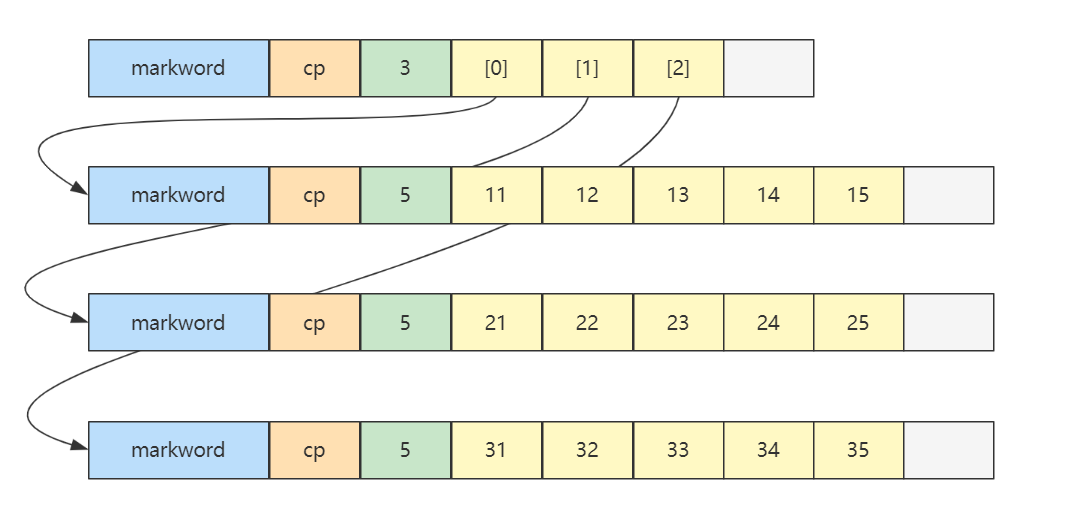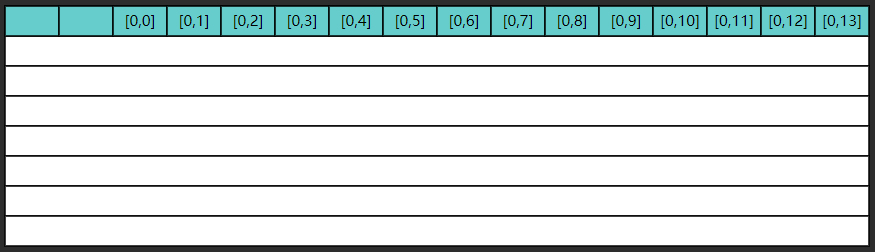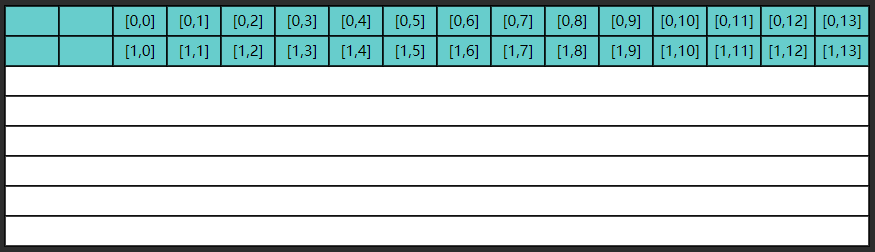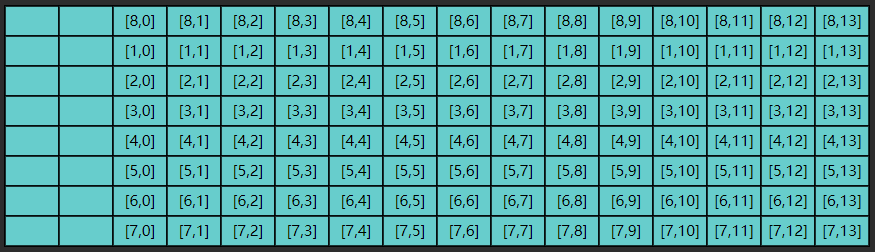• 概述
• 动态数组
• 二维数组
• 局部性原理
• 越界检查

# 概述

In computer science, an array is a data structure consisting of a collection of elements (values or variables), each identified by at least one array index or key

int[] array = {1,2,3,4,5}


• i i 即索引，在 Java、C 等语言都是从 0 开始
• s i z e size 是每个元素占用字节，例如 i n t int 4 4 d o u b l e double 8 8

byte[] array = {1,2,3,4,5}


Java 中数组结构为

• 8 字节 markword
• 4 字节 class 指针（压缩 class 指针的情况）
• 4 字节 数组大小（决定了数组最大容量是 2 32 2^{32}
• 数组元素 + 对齐字节（java 中所有对象大小都是 8 字节的整数倍[^12]，不足的要用对齐字节补足）

int[] array = {1, 2, 3, 4, 5};


8 + 4 + 4 + 5*4 + 4(alignment)


• 如果逻辑大小为0，数组为空，则说明该数组不包含数据项；
• 如果数组包含的数据项，数组最后一项的索引为逻辑大小减1；
• 如果逻辑大小等于物理大小，数组已经被数据填满。

# 动态数组

java 版本

import java.util.Arrays;
import java.util.Iterator;
import java.util.function.Consumer;
import java.util.stream.IntStream;

/**
* @author Ethan
* @date 2023/3/20
* @description
*/
public class Ds01DynamicArray implements Iterable<Integer> {
/**
*  逻辑大小
*/
private int size = 0;
/**
*  容量
*/
private int capacity = 8;
/**
* 初始化数组为空
*/
private int[] array = {};

/**
* 向任意位置添加元素
*
* @param index   索引位置
* @param element 待添加元素
*/
public void add(int index, int element) {
// 检查容量大小，不够要扩容
checkAndGrow();

// 如果插入的位置效益逻辑大小，那么要先把位置腾出来，索引位置以后得元素都要后移一位
if (index >= 0 && index < size) {
// 向后挪动, 空出待插入位置，使用数组的copy方法
// 从哪书分别是源数组、源数组起始位置、目标数组、目标数组的起始位置、copy元素个数
System.arraycopy(array, index,
array, index + 1, size - index);
}
// 在指定位置插入元素
array[index] = element;
// 逻辑大小+1
size++;
}

/**
* 向最后位置 [size] 添加元素
*
* @param element 待添加元素
*/
// 复用任意位置添加元素，插入位置是逻辑大小
}

/**
* 容量检查，不够进行扩容
*/
private void checkAndGrow() {
// 容量检查
if (size == 0) {
array = new int[capacity];
} else if (size == capacity) {
// 进行扩容, 1.5 1.618 2
capacity += capacity >> 1;
int[] newArray = new int[capacity];
System.arraycopy(array, 0,
newArray, 0, size);
array = newArray;
}
}

/**
* 从 [0 .. size) 范围删除元素
*
* @param index 索引位置
* @return 被删除元素
*/
public int remove(int index) { // [0..size)
// 要删除的元素
int removed = array[index];
// 如果要删除的元素索引小于逻辑大小-1，那么把目标索引的后面元素都向前移动一位
if (index < size - 1) {
// 向前挪动
System.arraycopy(array, index + 1,
array, index, size - index - 1);
}
// 逻辑大小-1
size--;
return removed;
}

/**
* 查询元素
*
* @param index 索引位置, 在 [0..size) 区间内
* @return 该索引位置的元素
*/
public int get(int index) {
return array[index];
}

/**
* 遍历方法1
*
* @param consumer 遍历要执行的操作, 入参: 每个元素
*/
public void foreach(Consumer<Integer> consumer) {
// 使用Consumer把拿到的元素交给调用者来使用，具体使用方法取决于调用者
for (int i = 0; i < size; i++) {
// 提供 array[i]
// 返回 void
consumer.accept(array[i]);
}
}

/**
* 遍历方法2 - 迭代器遍历，这个类要实现Iterator接口
*/
@Override
public Iterator<Integer> iterator() {
// 使用匿名内部类，直接返回一个迭代器，实现接口的两个方法
return new Iterator<Integer>() {
int i = 0;

@Override
public boolean hasNext() { // 有没有下一个元素
return i < size;
}

@Override
public Integer next() { // 返回当前元素,并移动到下一个元素
return array[i++];
}
};
}

/**
* 遍历方法3 - stream 遍历
*
* @return stream 流
*/
public IntStream stream() {
return IntStream.of(Arrays.copyOfRange(array, 0, size));
}

}

• 这些方法实现，都简化了 index 的有效性判断，假设输入的 index 都是合法的

**头部位置：**因为要把头部后面的元素都移动一位，所以时间复杂度是 O ( n ) O(n)

**中间位置：**一样要移动指定索引位置后的元素，所以时间复杂度是 O ( n ) O(n)

**尾部位置：**可直接通过索引找到最后一个元素，且不需要移动元素，所以时间复杂度是 O ( 1 ) O(1) （均摊来说）

# 二维数组

int[][] array = {
{11, 12, 13, 14, 15},
{21, 22, 23, 24, 25},
{31, 32, 33, 34, 35},
};• 最上面的二维数组占 32 个字节，其中 array，array，array 三个元素分别保存了指向三个一维数组的引用

• 三个一维数组各占 40 个字节

• 它们在内层布局上是连续

• m m 是外层数组的长度，可以看作 row 行
• n n 是内层数组的长度，可以看作 column 列
• 当访问 A r r a y [ i ] [ j ] Array[i][j] 0 ≤ i < m , 0 ≤ j < n 0\leq i \lt m, 0\leq j \lt n 时，就相当于
• 先找到第 i i 个内层数组（行）
• 再找到此内层数组中第 j j 个元素（列）

Java 环境下（不考虑类指针和引用压缩，此为默认情况），有下面的二维数组

byte[][] array = {
{11, 12, 13, 14, 15},
{21, 22, 23, 24, 25},
{31, 32, 33, 34, 35},
};


• 起始地址 0x1000
• 外层数组大小：16字节对象头 + 3元素 * 每个引用4字节 + 4 对齐字节 = 32 = 0x20
• 第一个内层数组大小：16字节对象头 + 5元素 * 每个byte1字节 + 3 对齐字节 = 24 = 0x18
• 第二个内层数组，16字节对象头 = 0x10，待查找元素索引为 2
• 最后结果 = 0x1000 + 0x20 + 0x18 + 0x10 + 2*1 = 0x104a

# 局部性原理

• cpu 读取内存（速度慢）数据后，会将其放入高速缓存（速度快）当中，如果后来的计算再用到此数据，在缓存中能读到的话，就不必读内存了
• 缓存的最小存储单位是缓存行（cache line），一般是 64 bytes，一次读的数据少了不划算啊，因此最少读 64 bytes 填满一个缓存行，因此读入某个数据时也会读取其临近的数据，这就是所谓空间局部性

int rows = 1000000;
int columns = 14;
int[][] a = new int[rows][columns];

StopWatch sw = new StopWatch();
sw.start("ij");
ij(a, rows, columns);
sw.stop();
sw.start("ji");
ji(a, rows, columns);
sw.stop();
System.out.println(sw.prettyPrint());


ij 方法

public static void ij(int[][] a, int rows, int columns) {
long sum = 0L;
for (int i = 0; i < rows; i++) {
for (int j = 0; j < columns; j++) {
sum += a[i][j];
}
}
System.out.println(sum);
}


ji 方法

public static void ji(int[][] a, int rows, int columns) {
long sum = 0L;
for (int j = 0; j < columns; j++) {
for (int i = 0; i < rows; i++) {
sum += a[i][j];
}
}
System.out.println(sum);
}


0
0
StopWatch '': running time = 96283300 ns
---------------------------------------------
---------------------------------------------
016196200  017%  ij
080087100  083%  ji


• 缓存是有限的，当新数据来了后，一些旧的缓存行数据就会被覆盖
• 如果不能充分利用缓存的数据，就会造成效率低下1. I/O 读写时同样可以体现局部性原理

2. 数组可以充分利用局部性原理，那么链表呢？

答：链表不行，因为链表的元素并非相邻存储

# 越界检查

java 中对数组元素的读写都有越界检查，类似于下面的代码

bool is_within_bounds(int index) const
{
return 0 <= index && index < length();
}

• 代码位置：openjdk\src\hotspot\share\oops\arrayOop.hpp